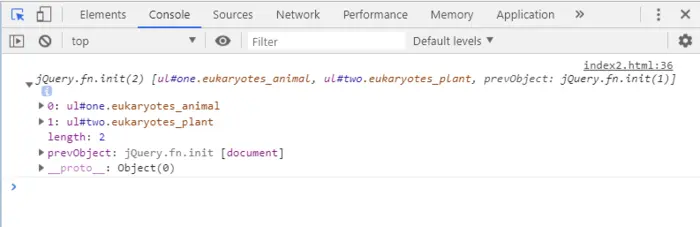# jquery中属性选择器是什么2023-03-10 19:15:23538浏览jquery 属性选择器介绍

jQuery属性选择器是基于元素属性作为筛选条件的选择器。

`<input id="btn" type="button" value="按钮" />`

jQuery属性选择器

E[attr]选择元素E，其中E元素必须带有attr属性
E[attr = “value”]选择元素E，其中E元素的attr属性取值是value
E[attr!= “value”]选择元素E，其中E元素的attr属性取值不是value
E[attr ^= “value”]选择元素E，其中E元素的attr属性取值是以“value”开头的任何字符
E[attr \$=“value”]选择元素E，其中E元素的attr属性取值是以“value”结尾的任何字符
E[attr *= “value”]选择元素E，其中E元素的attr属性取值是包含“value”的任何字符
E[attr |= “value”]选择元素E，其中E元素的attr属性取值等于“value”或者以“value”开头
E[attr ~= “value”]选择元素E，其中E元素的attr属性取值等于“value”或者包含“value”
[selector1][selector2][selectorN]多属性选择器(属性交集选择器)

jQuery这些属性选择器使得选择器具有通配符的功能，有点正则表达式的感觉。下面我们通过一些简单实例来认识一下。

`\$("div[class]")`

`\$("input[type = 'checkbox']")`

`\$("input[type != 'checkbox']")`

`\$("div[class *= 'nav']")`

```<div class="nav-header"></div>：
\$("div[class ^= 'nav']")```

```<div class="first-nav"></div>：
\$("div[class \$= 'nav']")```

```<div id="container" class="nav-header"></div>：
\$("div[id][class ^='nav']")```

```<!DOCTYPE style="color:rgb(73 238 255)">html>
<style="color:rgb(73 238 255)">html>

<style="color:rgb(73 238 255)">head lang="style="color:rgb(255 95 0)">zh-CN">
<style="color:rgb(73 238 255)">meta charset="style="color:rgb(255 95 0)">UTF-8">
<style="color:rgb(73 238 255)">meta name="style="color:rgb(98 189 255)">viewport" content="width=device-width,initial-scale=1,maximum-scale=1,user-scalable=no">
<style="color:rgb(255 95 0)">title>多项选择器</style="color:rgb(255 95 0)">title>
<style></style>

<body>
<section>
<ul id="style="color:rgb(255 111 119)">one" class="style="color:rgb(98 189 255)">eukaryotes_animal">
<li>猴子</li>
<li>猛犸</li>
<li>猩猩</li>
</ul>
<ul id="style="color:rgb(255 111 119)">two" class="style="color:rgb(98 189 255)">eukaryotes_plant">
<li>牡丹</li>
<li>樱花</li>
<li>仙人掌</li>
</ul>
<ul id='three' class="style="color:rgb(98 189 255)">prokaryotes_microbe">
<li>细菌</li>
<li>蓝细菌</li>
<li>放线菌</li>
<li>支原体</li>
</ul>

</section>
<script src="style="color:rgb(255 95 0)">https://style="color:rgb(255 111 119)">cdn.style="color:rgb(253 97 106)">bootcss.com/style="color:rgb(255 211 0)">jquery/3.3.1/style="color:rgb(255 211 0)">jquery.js"></script>
<script type="text/javascript">
//此处填写代码

});
</script>
</body>

</style="color:rgb(73 238 255)">html>```

[attribute] 属性名选择器

```var a=\$('[id]');
console.log(a);```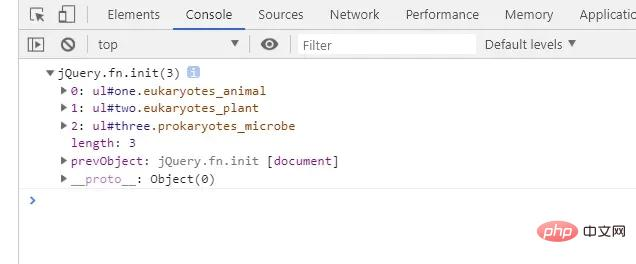[attribute=value]属性值选择器

```var a=\$('[id=one]');
console.log(a);```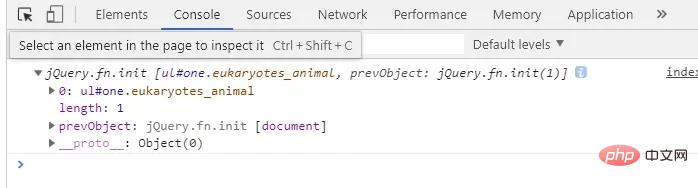[attribute!=value]非属性值选择器

```var a=\$('[class!=eukaryotes_animal]');
console.log(a);```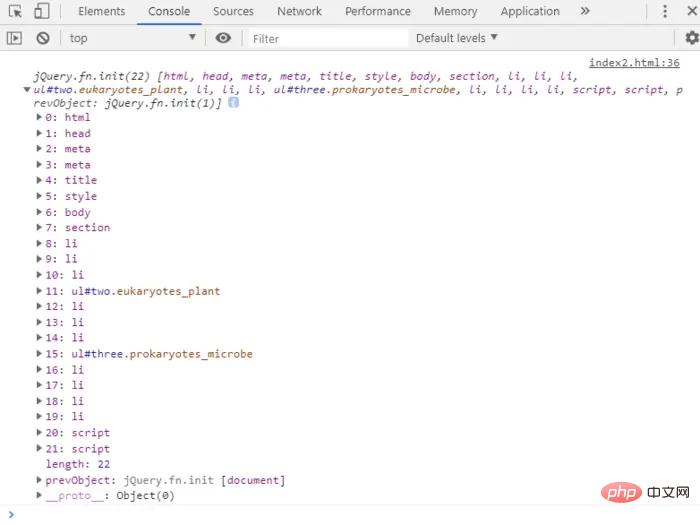[attribute^=value]属性值以某个字符串开头的选择器

```var a=\$('[class^=eukaryotes]');
console.log(a);```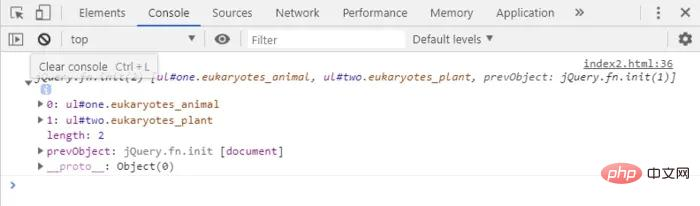[attribute\$=value]属性值以某个字符串结尾的选择器

```var a=\$('[class\$=plant]');
console.log(a);```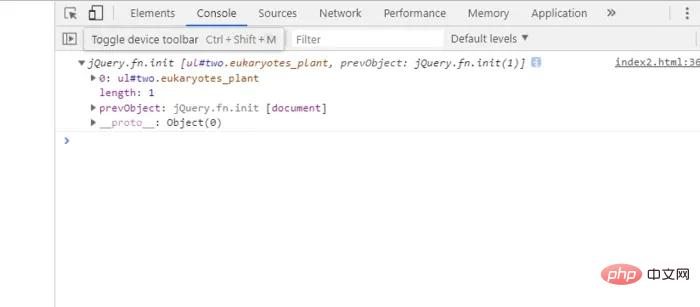[attribute*=value]属性值中包含某个字符串的选择器

```var a=\$('[class*=yotes_m]');
console.log(a);```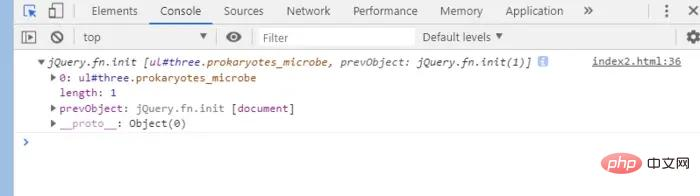[selector1][selector2][selectorN] 多属性选择器(属性交集选择器)

```var a=\$('[class^=eukaryotes_][id]');
console.log(a);```